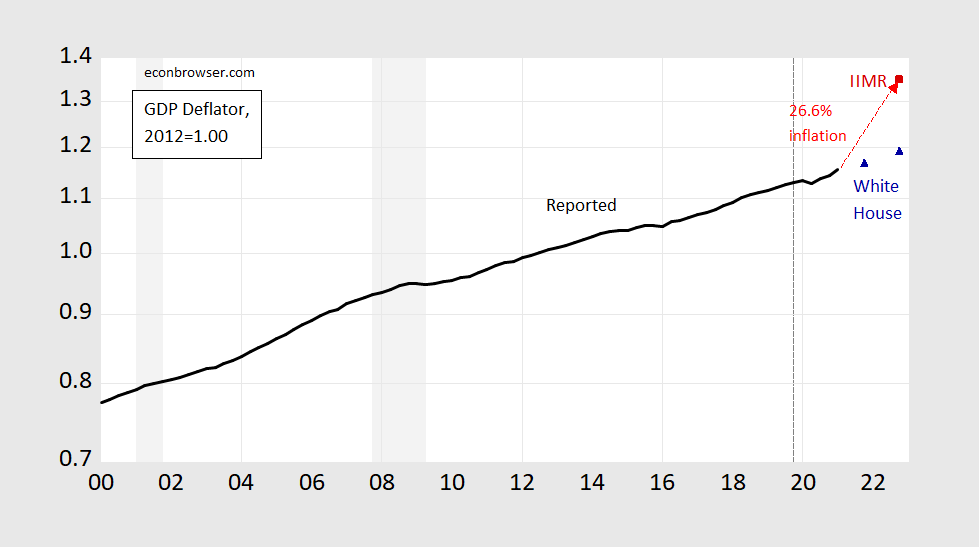## A Monetarist Manifesto For The Covid-19 Era

Juan E. Castañeda (Director) and Tim Congdon (Chairman) of the Institute for International Monetary Research at the University of Buckingham write today:

With the M3 velocity of circulation at 1.04, equilibrium nominal national income comes out as roughly \$29,000b.

…if nominal GDP needs to rise by 30% in a period of 18 months to two years, and if real output cannot go up in that period by more than, say, 8%, then the rest of the adjustment has to be seen in the price level. Continued boom between now and Q4 2022 in which real output rises by 8% and the price level by 20% would in fact result in a 30% jump in nominal GDP

Using the calculations implicit in their study, I derive the following graph of the GDP deflator (assuming 7% real growth by the end of 2022 ).Figure 1: US GDP price deflator (black), IIMR implied deflator as of 2022Q4 (red square), White House FY22 forecast implied deflator (blue triangle), all 2012=1 on log scale. IIMR calculation of price level assumes 7% growth. NBER defined recession dates shaded gray, dashed line at NBER peak date of 2019Q4. Source: BEA, 2021Q1 2nd release, IIMR (June 14, 2021), White House (May 2021), NBER, and author’s calculations.

As the authors note, the approach is essentially using the Quantity Theory, MV=PY. Use M3 for money (M), assume V (velocity) is mean reverting, obtain PY (nominal GDP), and then assume Y cannot rise more than a certain amount (7%, or 8%, depending on location in text). Mis a broader measure of money than M2   (post-2006 data sourced from Shadowstats). If my calculations are correct, this implies the GDP deflator will grow at 26.6% (annual rate) through 2022Q4. Over the 2000-2021Q1 period, the CPI has grown about 31% faster than the GDP deflator, so that suggests a CPI inflation rate of about 34.5% for the next 1.5 to 2 years.

Time will tell whether the Quantity Theory or some other approach (e.g., Phillips curve) proves more accurate. I discuss the past forecasting record of the Quantity Theory in this post.

Disclosure: None.